C Program to Find Common Elements in Two Array

In this tutorial you will learn about the C Program to Find Common Elements in Two Array and its application with practical example.

C Program to Find Common Elements in Two Array

In this tutorial, we will learn to create a C program that will Find Common Elements in Two Arrays using C programming.

Prerequisites

Before starting with this tutorial we assume that you are best aware of the following C programming topics:

• Operators in C Programming.
• Basic Input and Output function in C Programming.
• Basic C programming.
• For loop in C Programming.

Find Common Elements in Two Array:-

As we all know array is a collection of similar data type elements. In an array, only one variable is declared which can store multiple values. First will take the number of elements of an array from the user. Then will take the elements from the user for the array. And at last, we will Find Common Elements in Two Array C Programming Language.

Program:-

Find Common Elements in Two Array

Output:-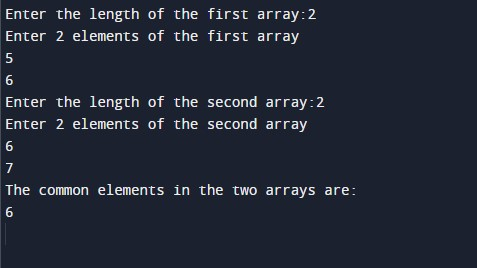In the above program, we have first initialized the required variable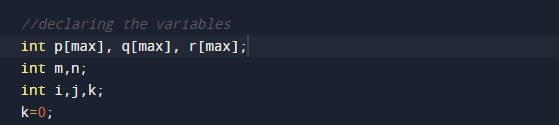• p[max] = it will hold the elements in an array.
• q[max] = it will hold the elements in an array.
• r[max] = it will hold the elements in an array.
• n = it will hold the number of elements in an array.
• m = it will hold the number of elements in an array.
• i = it will hold the integer value to control the array.
• j = it will hold the integer value to control the array.
• k = it will hold the integer value to control the array.

Taking input from the user in an array number of elements in the array.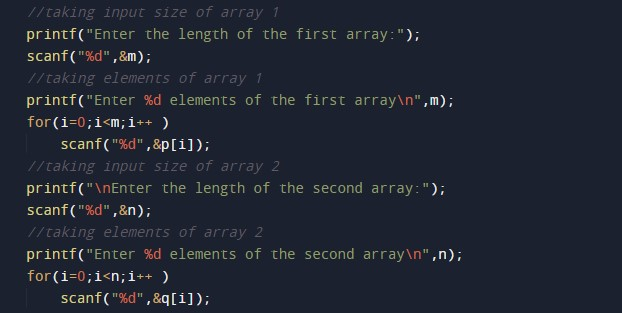Checking the array,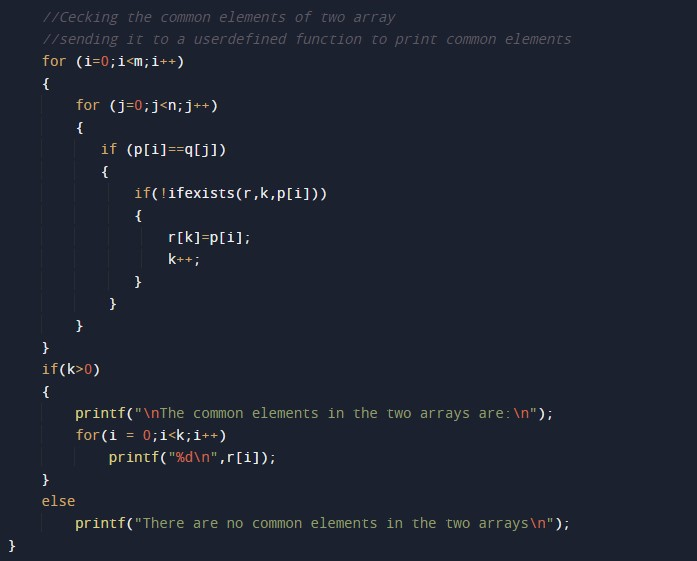Printing the results.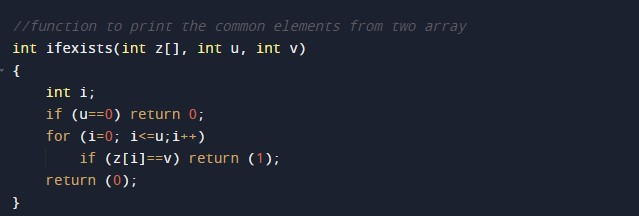In this tutorial we have learn about the C Program to Find Common Elements in Two Array and its application with practical example. I hope you will like this tutorial.